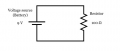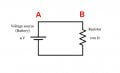# Rudimentary question for noob

Thread Starter

#### Totem71

Joined May 18, 2020
6
If, for example, had a hypothetical circuit with a 12 volt battery and a 100 ohm resistor in it. As the current circles, as it heat disappates thought the resistor and now a lower amount of current is flowing back to the battery - does the current lose any "power" and replenish while travelling back through the battery? Or does the voltage stay the same (assuming the voltage magically never runs out) except current has slown down? And as the current races by the resistor multiple times will the current ever eventually slow to a halt? I'm trying to understand the concept. Thank you!!!

#### MrChips

Joined Oct 2, 2009
23,541
Welcome to AAC!

This is a common misconception.
Current does not slow down. Voltage is not diminished

Current flows in a loop. What leaves the battery returns to the battery.
Ohm's Law applies,
Current I = V voltage / R resistance
In this example
V = 9V
R = 100Ω
I = 9V / 100Ω = 0.09A

A current of 0.09A flows through all the wires, the battery and the resistor.
Voltage is always a voltage difference, i.e. it is a potential difference between two points.
The voltage across the battery is 9V same as the voltage across the resistor (but in opposite direction).

In other words the total voltage across the battery must balance the total voltage cross the top wire, the resistor and the bottom wire.

In practice, the voltage across the top wire is zero, The voltage across the bottom wire is zero.
It doesn't really matter if the voltage across each wire was not zero, let us say for example 0.001V.
The sum of all three voltages, i.e. across the top wire, across the resistor, plus across the bottom wire, must be equal to the voltage across the battery.
If this were the case, the voltage across the resistor must be 8.998V in order to balance the equation.
And the current will no longer be 0.09A!
See if you can determine the current now using Ohm's Law.Thread Starter

#### Totem71

Joined May 18, 2020
6
Welcome to AAC!

This is a common misconception.
Current does not slow down. Voltage is not diminished

Current flows in a loop. What leaves the battery returns to the battery.
Ohm's Law applies,
Current I = V voltage / R resistance
In this example
V = 9V
R = 100Ω
I = 9V / 100Ω = 0.09A

A current of 0.09A flows through all the wires, the battery and the resistor.
Voltage is always a voltage difference, i.e. it is a potential difference between two points.
The voltage across the battery is 9V same as the voltage across the resistor (but in opposite direction).

In other words the total voltage across the battery must balance the total voltage cross the top wire, the resistor and the bottom wire.

In practice, the voltage across the top wire is zero, The voltage across the bottom wire is zero.
It doesn't really matter if the voltage across each wire was not zero, let us say for example 0.001V.
The sum of all three voltages, i.e. across the top wire, across the resistor, plus across the bottom wire, must be equal to the voltage across the battery.
If this were the case, the voltage across the resistor must be 8.998V in order to balance the equation.

View attachment 207515

Thread Starter

#### Totem71

Joined May 18, 2020
6
Thank you for such a quick reply! Let me ask this - I run a marathon with a 100 other people (powered by a battery). We encounter "resistance", park benches, cars etc. Slows us down. But we complete the circuit. But we arent as fast as we were at the beginning. So we start again but at the start "voltage" line we are suddenly ready to do it again. If I build a circuit with 9 different resistors, how can the charged electrons not be slowed completely until it reaches that battery I started it with?

Thread Starter

#### Totem71

Joined May 18, 2020
6
As well, how can there be a voltage difference if voltage in = voltage out?

#### MrChips

Joined Oct 2, 2009
23,541
Because electrons are not people. You cannot use that analogy.

Thread Starter

#### Totem71

Joined May 18, 2020
6
I think I may be getting this - the current from say a 12vdc battery will always flow the same rate until the battery dies. But the voltage will change because the resistor slows the current resulting in a loss of voltage which is renewed at the source. I sure hope that is true because to me that makes perfect sense.

#### Delta Prime

Joined Nov 15, 2019
1,022
Let me ask this - I run a marathon with a 100 other people (powered by a battery). We encounter "resistance", park benches, cars etc. Slows us down
mr. Chips beat me to it you can't use that analogy.#### MrChips

Joined Oct 2, 2009
23,541
As well, how can there be a voltage difference if voltage in = voltage out?
Let us take the top wire as an example.
The left-hand side of the wire we will label as point-A.
The right-hand side of the wire we will label as point-B.Our first impression is the voltage at point-A is 9V. The voltage at point-B is 9V.
The voltage difference from point-A to point-B is 0V.
According to Ohm's Law I = V /R, the current must be zero because V = 0.
This contradicts what I said previously that the current is 0.09A.

Hah!
That is because the voltage at point-B is not 9V. It is 8.999999V. (enter as many 9s as you please).
That must also mean that the resistance of the wire is not 0Ω.
See if you can determine the resistance of the top wire using Ohm's Law.
(Bear in mind that no piece of wire has zero resistance, even super-conducting wires.)

#### MrChips

Joined Oct 2, 2009
23,541
I think I may be getting this - the current from say a 12vdc battery will always flow the same rate until the battery dies. But the voltage will change because the resistor slows the current resulting in a loss of voltage which is renewed at the source. I sure hope that is true because to me that makes perfect sense.
Depends on the battery.
If the battery is a fresh fully charged automotive battery you will be waiting for a very long time to see any change.

The voltage does not fall because the current slows down. The voltage falls because of changing chemistry taking place in the battery.

Thread Starter

#### Totem71

Joined May 18, 2020
6
Thank you very much! That was helpful.

#### Delta Prime

Joined Nov 15, 2019
1,022
Man you guys are too fast. I wanted to play now it's over thanks.Similar threads